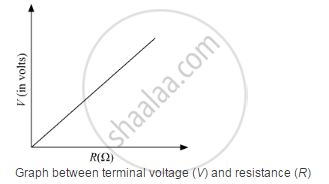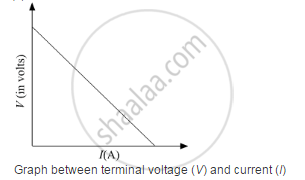Share

# A Cell of Emf 'E' and Internal Resistance 'r' is Connected Across a Variable Load Resistor R. Draw the Plots of the Terminal Voltage V Versus (I) R and (Ii) the Current I. - CBSE (Science) Class 12 - Physics

#### Question

A cell of emf 'E' and internal resistance 'r' is connected across a variable load resistor R. Draw the plots of the terminal voltage V versus (i) R and (ii) the current I.

It is found that when R = 4 Ω, the current is 1 A and when R is increased to 9 Ω, the current reduces to 0.5 A. Find the values of the emf E and internal resistance r.

#### Solution

(i)(ii)When R = 4 Ω and I = 1 A

We know

Terminal voltage, V = EIr

So, we have

V = IR = 4 = E − Ir

or

E − r = 4   ..... (i)

When R' = 9 Ω and I' = 0.5 A

V = IR = 0.5 × 9 = E − 0.5r

or

E − 0.5r = 4.5   ..... (ii)

Solving (i) and (ii), we get

r = 1 Ω and E = 5 V

Is there an error in this question or solution?

#### Video TutorialsVIEW ALL 

Solution A Cell of Emf 'E' and Internal Resistance 'r' is Connected Across a Variable Load Resistor R. Draw the Plots of the Terminal Voltage V Versus (I) R and (Ii) the Current I. Concept: Cells, Emf, Internal Resistance.
S